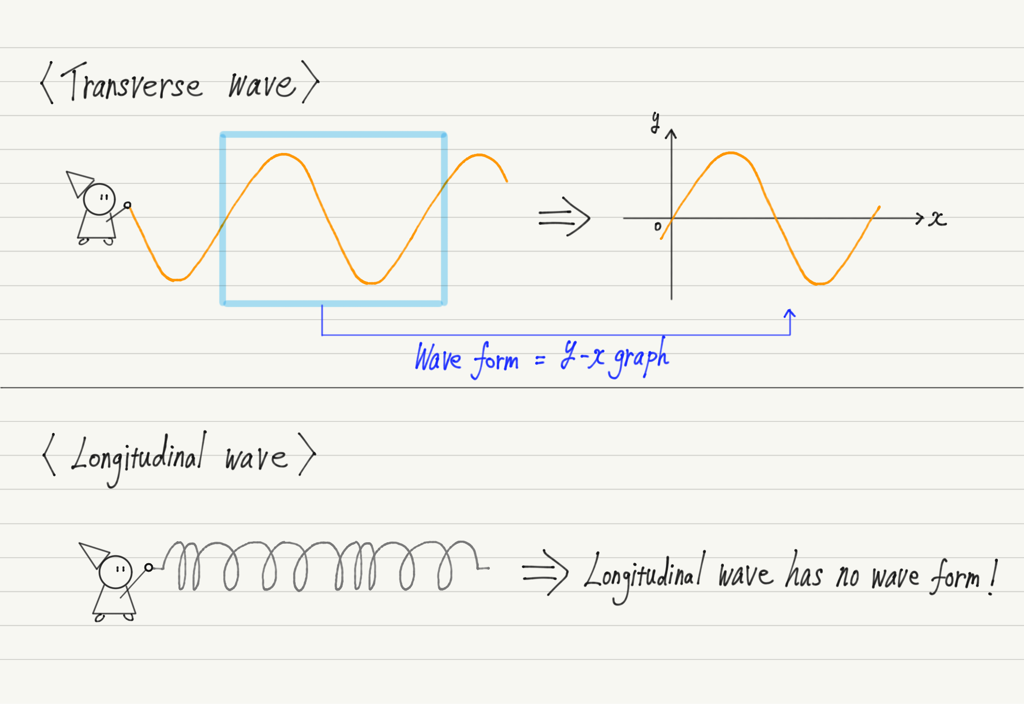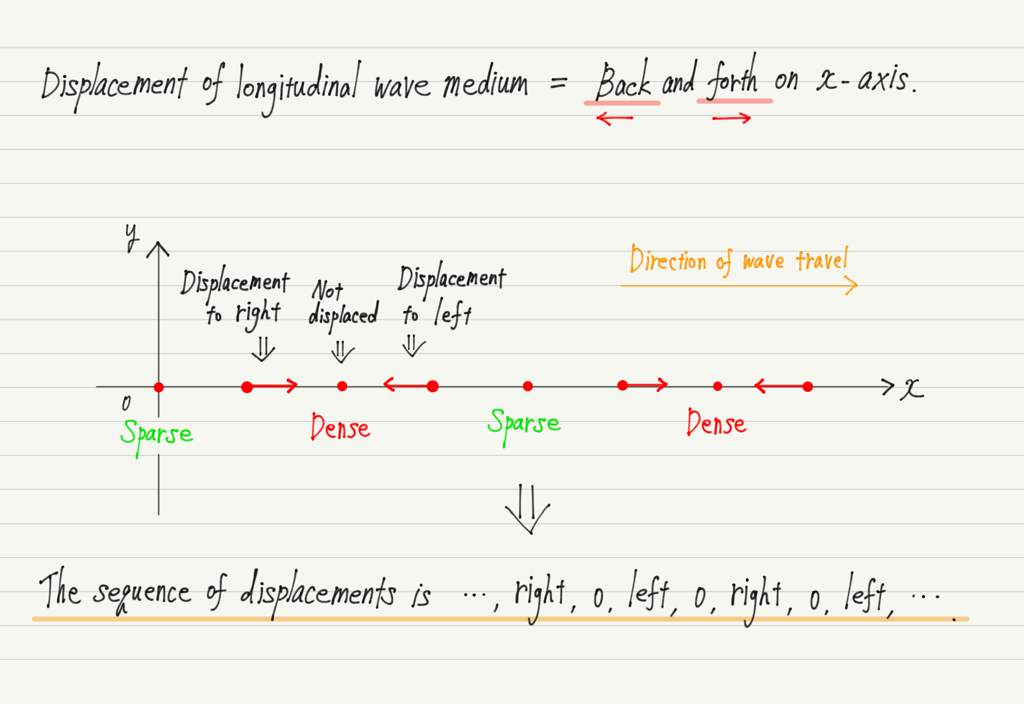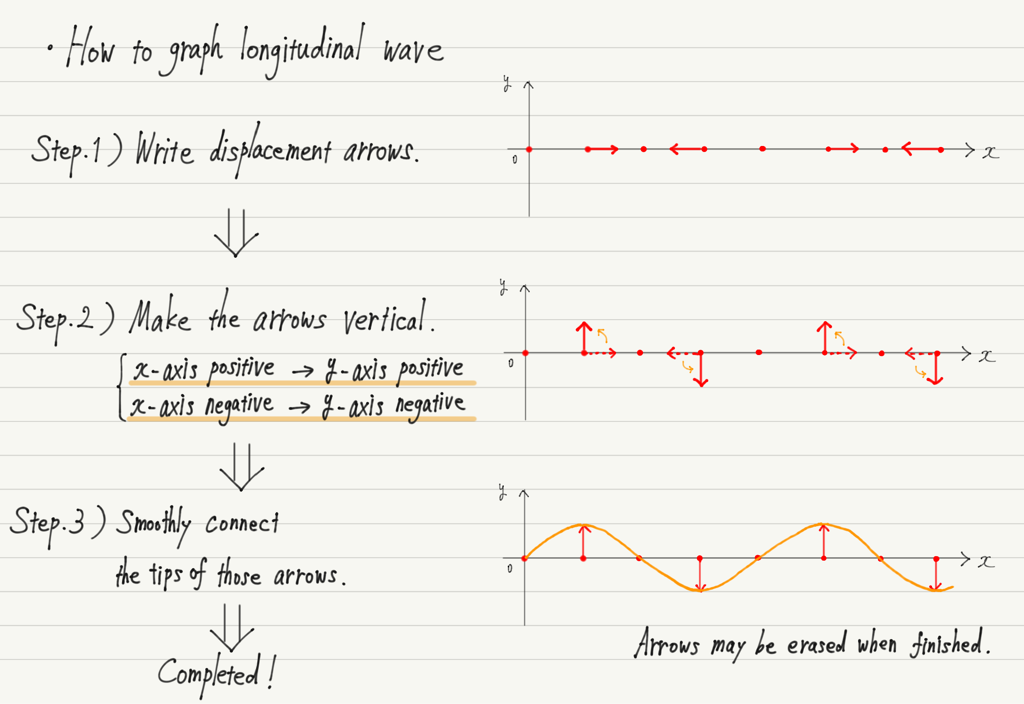# Graph of Longitudinal Wave

(This article was translated from Japanese to English using DeepL.)

Do you remember when we studied graphs of waves in the past, we used a rope as an example?
Waves transmitted by swinging a rope are transverse waves, so we were explaining the graph of a transverse wave at that time.

So what does a graph of a longitudinal wave look like?

## How to graph longitudinal wave

In a transverse wave, each point of the medium oscillates perpendicular to the direction of the wave, so the waveform is a sine wave as it is.

On the other hand, a longitudinal wave oscillates parallel to the direction of the wave, so the shape of the sine wave is not visible (the reason why longitudinal waves are difficult to visualize).So let’s look at the displacement of each point in the medium!
In a longitudinal wave, the medium moves back and forth in the direction of the wave, which is represented by an arrow.As shown in the figure above, there will be a point moving in the positive direction of the x-axis, a point moving in the negative direction, and a point that does not move.

Here, the points flanked by arrows (→・←) are dense because they are compressed from both sides. Conversely, the points where the arrows move away from each other in both directions (←・→) are sparse.

This arrowed representation of the displacement of the medium shows where the density and sparsity are located. If you have forgotten about dense and sparse, review the previous lecture.

https://www.yukimura-physics.com/entry/wave-f08

However, just lining up arrows is not enough to call it a graph, is it?
So, in order to draw a graph, we will modify these arrows!
Specifically, “an arrow along the x-axis is directed toward the y-axis.”
(This is just a graphical contrivance, it has no physical significance.)

If the displacement is along the y-axis, it is the same as a transverse wave and can be plotted on a graph.

Let me show you the actual procedure for drawing a graph.Now we can represent the longitudinal wave on a graph!

## Next Time

In the next lecture, we will learn about the characteristic properties of waves!

https://www.yukimura-physics.com/entry/wave-f10

error: Content is protected !!